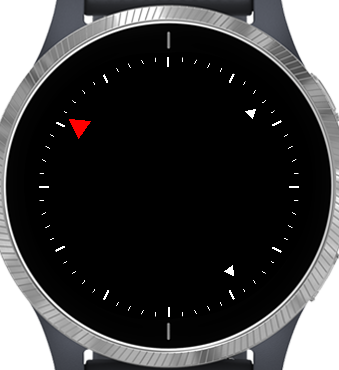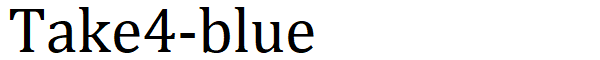# Creating a Garmin watch face: performance 2

Creating a Garmin watch face: performance as a reference, let’s take a quick look at what kind of implementation could improve performance.

## Watch face to measure

This time, I used an analog watch face that I was working on.The hour hand is red and the minute and second hands are white triangles.
A scale drawn around the screen. On the scale, the portion representing the time was drawn longer with a width of 2 dots, and the time was divided into 5 portions.

## Result

For the time being, the results measured by the following program only change the execution part, and there is no change in the time of the graphic drawing and display part. Therefore, only the time of the execution part is described.

The variable center in the program represents the center coordinates of the clock.

### Program 1

There was a feeling about 20 years ago, and I first saw a program that reduced the amount of computation to find sin / cos. In the past, sin / cos calculations were slower than the four arithmetic operations.
So was the addition theorem used to calculate the coordinates of the triangle vertices and the start and end points of the scale? I tried to calculate only the sin / cos of the variation angle, and then calculate the coordinates by four arithmetic operations.
The following is an excerpt of only the part where the scale is drawn.

``````// Create a sequence of points from the start position with the center as the center and the angle as the variation angle
// Feeling to make a point on the circumference around the center
class DeltaPosition {
var vSin;
var vCos;
var centerPosition;
var position;

function initialize(angle, center, start) {
vSin = Math.sin(angle);
vCos = Math.cos(angle);
centerPosition = center;
position = start;
}

function setPosition(start) {
position = start;
}

// Extract point on current circle
function getPosition() {
return [centerPosition + position, centerPosition + position];
}

// extract the point on the circumference moved by angle
function next() {
position = [position * vCos - position * vSin, position * vSin + position * vCos];
return getPosition();
}
}
// Generate coordinates and display ticks using variant angles
// Generate angles use the DeltaPosition class
function drawHashMarks(dc) {
var vS = new DeltaPosition(Math.PI / 30.0, center, [0, 0 - center]);
var vEl = new DeltaPosition(Math.PI / 30.0, center, [0, 10 - center]);	// hour mark
var vEs = new DeltaPosition(Math.PI / 30.0, center, [0, 5 - center]);	// 12 minute mark

dc.setPenWidth(2);
dc.drawLine(vS.getPosition(), vS.getPosition(), vEl.getPosition(), vEl.getPosition());
for (var i = 1; i < 60; ++i) {
var vNS = vS.next();
var vNEl = vEl.next();
var vNEs = vEs.next();
if (i % 5 == 0) {
dc.setPenWidth(2);
dc.drawLine(vNS, vNS, vNEl, vNEl);
}
else {
dc.setPenWidth(1);
dc.drawLine(vNS, vNS, vNEs, vNEs);
}
}
}
``````

The result of this implementation is 131ms.

### Program 2

As a comparison object, a method of calculating sin / cos every time from the angle of the scale was created as a method of calculating the coordinates of the scale. Similarly, only the part where the scale is drawn is extracted.

``````    function drawHashMarks1(dc) {
for (var i = 0; i < 60; ++i) {
var vSin = Math.sin(Math.PI / 30.0 * i);
var vCos = Math.cos(Math.PI / 30.0 * i);
if (i % 5 == 0) {
dc.setPenWidth(2);
dc.drawLine(center + center * vSin, center + center * vCos,
center + (center - 10) * vSin, center + (center - 10) * vCos);
}
else {
dc.setPenWidth(1);
dc.drawLine(center + center * vSin, center + center * vCos,
center + (center - 5) * vSin, center + (center - 5) * vCos);
}
}
}

``````

The result of this implementation is 51ms. Obviously less than program 1.

### Program 3

The differences between Program 1 and Program 2 are significant, so the part that seems to be slow in Program 1 first. I thought that it might be necessary to renew the DeltaPosition class used to construct the coordinates, so I tried to change this part to non-new code.

This is the idea of a long time ago, because I thought that freeing up memory would be quite expensive.

``````    function drawHashMarks2(dc) {
var position = [[0, 0 - center], [0, 10 - center], [0, 5 - center]];
var vSin = Math.sin(Math.PI / 30.0);
var vCos = Math.cos(Math.PI / 30.0);

dc.setPenWidth(2);
dc.drawLine(center + position, center + position,
center + position, center + position);
for (var i = 1; i < 60; ++i) {
for (var j = 0; j < 3; ++j) {
position[j] = [position[j] * vCos - position[j] * vSin, position[j] * vSin + position[j] * vCos];
}
if (i % 5 == 0) {
dc.setPenWidth(2);
dc.drawLine(center + position, center + position,
center + position, center + position);
}
else {
dc.setPenWidth(1);
dc.drawLine(center + position, center + position,
center + position, center + position);
}
}
}

``````

The result of this implementation is 93ms. It is faster than program 1, but slower than program 2.

## Discussion of results so far

The number of sin / cos is not slowed down, and memory management such as new did not slow down dramatically, so here is the faster the simpler the number of operations is?

### Program 4

This is an implementation that reduces the number of calculations based on Program 2.
`Math.PI / 30.0` used for calculating the angle of sin / cos, and the calculation of the length of the major and minor ticks outside the for statement.

``````    // Generate coordinates and display ticks using absolute angle values
function drawHashMarks1(dc) {
var vLong = center - 10;
var vShort = center - 5;
var aDel = Math.PI / 30.0;
for (var i = 0; i < 60; ++i) {
var vAngle = aDel * i;
var vSin = Math.sin(vAngle);
var vCos = Math.cos(vAngle);
if (i % 5 == 0) {
dc.setPenWidth(2);
dc.drawLine(center + center * vSin, center + center * vCos,
center + vLong * vSin, center + vLong * vCos);
}
else {
dc.setPenWidth(1);
dc.drawLine(center + center * vSin, center + center * vCos,
center + vShort * vSin, center + vShort * vCos);
}
}
}
``````

The result of this implementation is 43ms. 8 ms ahead of 51 ms in program 2.

## Anyway conclusion

As far as this program is concerned, regarding the execution processing part,

• It is necessary to keep the number of processes as small as possible, including calling functions, etc.

Does that mean?

Monkey C, unlike C # or C ++, does not optimize programs by compiling or linking, so you have to optimize yourself.
Maybe it’s the same interpreted language as Python.